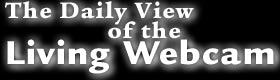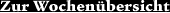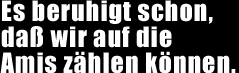14. November 2000one two three four five six seven eight nine ten and again one two three four five six seven eight nine ten and again one two three four five six seven eight nine ten and again one two three four five six seven eight nine ten and again one two three four five six seven eight nine ten and again one two three four five six seven eight nine ten and again one two three four five six seven eight nine ten and again one two three four five six seven eight nine ten and again one two three four five six seven eight nine ten and again one two three four five six seven eight nine ten and again one two three four five six seven eight nine ten and again one two three four five six seven eight nine ten and again one two three four five six seven eight nine ten and again one two three four five six seven eight nine ten and again one two three four five six seven eight nine ten and again one two three four five six seven eight nine ten and again one two three four five six seven eight nine ten and again one two three four five six seven eight nine ten and again one two three four five six seven eight nine ten and again one two three four five six seven eight nine ten and again one two three four five six seven eight nine ten and again one two three four five six seven eight nine ten and again one two three four five six seven eight nine ten and again one two three four five six seven eight nine ten and again one two three four five six seven eight nine ten and again one two three four five six seven eight nine ten and again one two three four five six seven eight nine ten and again one two three four five six seven eight nine ten and again one two three four five six seven eight nine ten and again one two three four five six seven eight nine ten and again one two three four five six seven eight nine ten and again ...